### Lecture 6:¶

• get a first peek at the very useful Python packages called NumPy and matplotlib

In the last lecture we learned how to create modules. These are files that contain one or more functions and variables. They can be imported and used in other programs or notebooks, saving us a lot of time and headache.

A Python package contains a collection of modules that are related to each other. Our first package is one of the most useful ones for us science types: NumPy.

### A first look at NumPy¶

O.K. First of all - how do you pronounce "NumPy"'? It should be pronounced "Num" as in "Number" and "Pie" as in, well, pie, or Python. It is way more fun to say Numpee! I try to suppress this urge.

Now with that out of the way, what can we do with NumPy? Turns out, a whole heck of a lot! But for now, we will just scratch the surface. For starters, NumPy can give us the value of the square root of a number with the function numpy.sqrt( ). Note how the package name comes first, then the function we wish to use (just as in our example from the last lecture).

To use NumPy functions, we must first import the package with the command import. It may take a while the first time you use import after installing Python, but after that it should load quickly.

We encountered import very briefly in the last lecture. Now it is time to go deeper. There are many different ways you can use the import command. Each way allows your program to access the functions and variables defined in the imported package, but differs in how you call the function after importing:

In :
import numpy

#This makes all the functions in NumPy available to you,
#but you have to call them with the numpy.FUNC() syntax

numpy.sqrt(2)

Out:
1.4142135623730951
In :
# Here is another way to import a module:
import numpy as np  # or any other variable e.g.:  N
# This does the same as the first, but allows you to set NumPy with a nickname

# In this case, you substitute "np" for numpy:

np.sqrt(2)  # or N.pi in the second case.

# Note: Some folks in the NumPy community use N; I use np.
# That seems to be the most common way now.

Out:
1.4142135623730951

To import all the functions from NumPy:

In :
from numpy  import *

# now all the functions are available directly, without the initial module name:

sqrt(2)

Out:
1.4142135623730951

The '*' imports all the functions into the local namespace, which is a heavy load on your memory. Alternatively, you can import the few, specific functions you'll use, for example, sqrt:

In :
from numpy import sqrt # square root

sqrt(4)

Out:
2.0

Did you notice how "sqrt(4)", where 4 was an integer, returned a floating point variable (2.0)?

TIP: I tend to import the NumPy package using the np option above. That way I know where the functions I'm using come from. This is useful, becuase we don't use or know ALL of the functions available in any given package. AND the same function name can mean different things in different packages. So, a function defined in the package could conflict with one defined in your program. It is just good programming practice to specify the origin of the function you are using.

### NumPy functions¶

Here is a (partial) list of some useful NumPy functions:

function purpose
absolute(x) absolute value
arccos(x) arccosine
arcsin(x) arcsine
arctan(x) arctangent
arctan2(y,x) arctangent of y/x in correct quadrant
cos(x) cosine
cosh(x) hyperbolic cosine
exp(x) exponential
log(x) natural logarithm
log10(x) base 10 log
sin(x) sine
sinh(x) hyperbolic sine
sqrt(x) square root
tan(x) tangent
tanh(x) hyperbolic tangent

### Numpy attributes¶

NumPy has more than just functions; it also has attributes which are variables stored in the package, for example $\pi$.

In :
np.pi

Out:
3.141592653589793

TIP: In the trigonometric functions, the argument is in RADIANS!.You can convert between degrees and radians by multiplying by: np.pi/180. OR you can convert using the NumPy functions np.degrees( ) which converts radians to degrees and np.radians( ) which converts degrees to radians.

Also notice how the functions have parentheses, as opposed to np.pi which does not. The difference is that np.pi is not a function but an attribute. It is a variable defined in NumPy that you can access. Every time you call the variable np.pi, it returns the value, $\pi$.

### Using NumPy Functions¶

As already mentioned, NumPy has many math functions. We will use a few to generate some data sets that we can then plot using matplotlib, a different Python module.

First, let's make a list of angles ($\theta$ or theta) around a circle. We begin with the list of angles in degrees, convert them to radians (using np.radians( )), then construct a list of sines of those angles.

In :
thetas_in_degrees=range(0,360,5) # list (generator) of angles from 0 to 359 at five degree intervals
# uncomment the following line, if you'd like to print the list
#print (list(thetas_in_degrees))
sines=np.sin(thetas_in_radians) # calculate the sine values for all the thetas
sines

Out:
array([ 0.00000000e+00,  8.71557427e-02,  1.73648178e-01,  2.58819045e-01,
3.42020143e-01,  4.22618262e-01,  5.00000000e-01,  5.73576436e-01,
6.42787610e-01,  7.07106781e-01,  7.66044443e-01,  8.19152044e-01,
8.66025404e-01,  9.06307787e-01,  9.39692621e-01,  9.65925826e-01,
9.84807753e-01,  9.96194698e-01,  1.00000000e+00,  9.96194698e-01,
9.84807753e-01,  9.65925826e-01,  9.39692621e-01,  9.06307787e-01,
8.66025404e-01,  8.19152044e-01,  7.66044443e-01,  7.07106781e-01,
6.42787610e-01,  5.73576436e-01,  5.00000000e-01,  4.22618262e-01,
3.42020143e-01,  2.58819045e-01,  1.73648178e-01,  8.71557427e-02,
1.22464680e-16, -8.71557427e-02, -1.73648178e-01, -2.58819045e-01,
-3.42020143e-01, -4.22618262e-01, -5.00000000e-01, -5.73576436e-01,
-6.42787610e-01, -7.07106781e-01, -7.66044443e-01, -8.19152044e-01,
-8.66025404e-01, -9.06307787e-01, -9.39692621e-01, -9.65925826e-01,
-9.84807753e-01, -9.96194698e-01, -1.00000000e+00, -9.96194698e-01,
-9.84807753e-01, -9.65925826e-01, -9.39692621e-01, -9.06307787e-01,
-8.66025404e-01, -8.19152044e-01, -7.66044443e-01, -7.07106781e-01,
-6.42787610e-01, -5.73576436e-01, -5.00000000e-01, -4.22618262e-01,
-3.42020143e-01, -2.58819045e-01, -1.73648178e-01, -8.71557427e-02])

### Plotting data¶

Now that we've generated some data, we can look at them. Yes, we just printed out the values, but it is way more interesting to make a plot. The easiest way to do this is using the package matplotlib which has many plotting functions, among them a whole module called pyplot. By convention, we import the matplotlib.pyplot module as plt.

We've also included one more line that tells pyplot to plot the image within the notebook: The magic command: %matplotlib inline. Note that this does not work in other environments, like command line scripts; magic commands are only for Jupyter notebooks.

In :
import matplotlib.pyplot as plt # import the plotting module
# call this magic command to show the plots in the notebook
%matplotlib inline

plt.plot(thetas_in_degrees,sines); # plot the sines with the angles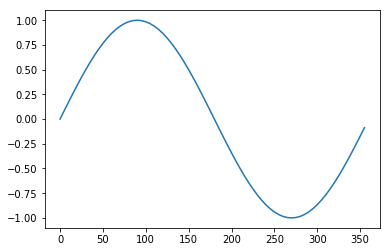### Features and styling in matplotlib¶

Every plot should at least have axis labels and can also have a title, a legend, bounds, etc. We can use matplotlib.pyplot to add these features and more.

In :
# I want to plot the sine curve as a green line, so I use 'g-' to do that:
plt.plot(thetas_in_degrees,sines,'g-',label='Sine')
# the "label" argument saves this line for annotation in a legend
# let's add X and Y labels
plt.xlabel('Degrees') # make and X label
plt.ylabel('Sine') # label the Y axis
# and now change the x axis limits:
plt.xlim([0,360]) # set the limits
plt.title('Sine curve') # set the title
plt.legend(); # put on a legend!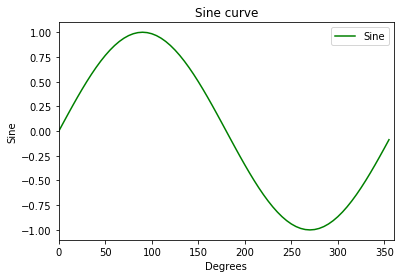Now let's add the cosine curve and a bit of style! We'll plot the cosine curve as a dashed blue line ('b--'), move the legend to a different position and plot the sine curve as little red dots ('r.'). For a complete list of possible symbols (markers), see: http://matplotlib.org/api/markers_api.html

In :
cosines=np.cos(thetas_in_radians)
# plot the sines with the angles as a green line
plt.plot(thetas_in_degrees,sines,'r.',label='Sine')
# plot the cosines with the angles as a dashed blue line
plt.plot(thetas_in_degrees,cosines,'b--',label='Cosine')
plt.xlabel('Degrees')
plt.ylabel('Trig functions')
plt.xlim([0,360]) # set the limits
plt.legend(loc=3); # put the legend in the lower left hand corner this time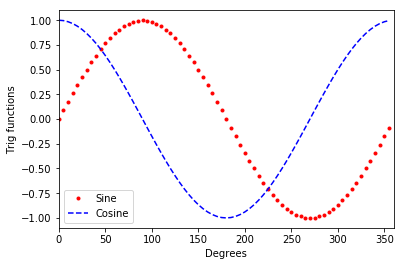The function plt.plot( ) in matplotlib.pyplot includes many more styling options. Here's a complete list of arguments and keyword arguments that plot accepts:

In :
help(plt.plot)

Help on function plot in module matplotlib.pyplot:

plot(*args, **kwargs)
Plot y versus x as lines and/or markers.

Call signatures::

plot([x], y, [fmt], data=None, **kwargs)
plot([x], y, [fmt], [x2], y2, [fmt2], ..., **kwargs)

The coordinates of the points or line nodes are given by *x*, *y*.

The optional parameter *fmt* is a convenient way for defining basic
formatting like color, marker and linestyle. It's a shortcut string
notation described in the *Notes* section below.

>>> plot(x, y)        # plot x and y using default line style and color
>>> plot(x, y, 'bo')  # plot x and y using blue circle markers
>>> plot(y)           # plot y using x as index array 0..N-1
>>> plot(y, 'r+')     # ditto, but with red plusses

You can use .Line2D properties as keyword arguments for more
control on the  appearance. Line properties and *fmt* can be mixed.
The following two calls yield identical results:

>>> plot(x, y, 'go--', linewidth=2, markersize=12)
>>> plot(x, y, color='green', marker='o', linestyle='dashed',
linewidth=2, markersize=12)

When conflicting with *fmt*, keyword arguments take precedence.

**Plotting labelled data**

There's a convenient way for plotting objects with labelled data (i.e.
data that can be accessed by index obj['y']). Instead of giving
the data in *x* and *y*, you can provide the object in the *data*
parameter and just give the labels for *x* and *y*::

>>> plot('xlabel', 'ylabel', data=obj)

All indexable objects are supported. This could e.g. be a dict, a
pandas.DataFame or a structured numpy array.

**Plotting multiple sets of data**

There are various ways to plot multiple sets of data.

- The most straight forward way is just to call plot multiple times.
Example:

>>> plot(x1, y1, 'bo')
>>> plot(x2, y2, 'go')

- Alternatively, if your data is already a 2d array, you can pass it
directly to *x*, *y*. A separate data set will be drawn for every
column.

Example: an array a where the first column represents the *x*
values and the other columns are the *y* columns::

>>> plot(a, a[1:])

- The third way is to specify multiple sets of *[x]*, *y*, *[fmt]*
groups::

>>> plot(x1, y1, 'g^', x2, y2, 'g-')

In this case, any additional keyword argument applies to all
datasets. Also this syntax cannot be combined with the *data*
parameter.

By default, each line is assigned a different style specified by a
'style cycle'. The *fmt* and line property parameters are only
necessary if you want explicit deviations from these defaults.
Alternatively, you can also change the style cycle using the
'axes.prop_cycle' rcParam.

Parameters
----------
x, y : array-like or scalar
The horizontal / vertical coordinates of the data points.
*x* values are optional. If not given, they default to
[0, ..., N-1].

Commonly, these parameters are arrays of length N. However,
scalars are supported as well (equivalent to an array with
constant value).

The parameters can also be 2-dimensional. Then, the columns
represent separate data sets.

fmt : str, optional
A format string, e.g. 'ro' for red circles. See the *Notes*
section for a full description of the format strings.

Format strings are just an abbreviation for quickly setting
basic line properties. All of these and more can also be
controlled by keyword arguments.

data : indexable object, optional
An object with labelled data. If given, provide the label names to
plot in *x* and *y*.

.. note::
Technically there's a slight ambiguity in calls where the
second label is a valid *fmt*. plot('n', 'o', data=obj)
could be plt(x, y) or plt(y, fmt). In such cases,
the former interpretation is chosen, but a warning is issued.
You may suppress the warning by adding an empty format string
plot('n', 'o', '', data=obj).

Other Parameters
----------------
scalex, scaley : bool, optional, default: True
These parameters determined if the view limits are adapted to
the data limits. The values are passed on to autoscale_view.

**kwargs : .Line2D properties, optional
*kwargs* are used to specify properties like a line label (for
auto legends), linewidth, antialiasing, marker face color.
Example::

>>> plot([1,2,3], [1,2,3], 'go-', label='line 1', linewidth=2)
>>> plot([1,2,3], [1,4,9], 'rs',  label='line 2')

If you make multiple lines with one plot command, the kwargs
apply to all those lines.

Here is a list of available .Line2D properties:

agg_filter: a filter function, which takes a (m, n, 3) float array and a dpi value, and returns a (m, n, 3) array
alpha: float (0.0 transparent through 1.0 opaque)
animated: bool
antialiased or aa: bool
clip_box: a .Bbox instance
clip_on: bool
clip_path: [(~matplotlib.path.Path, .Transform) | .Patch | None]
color or c: any matplotlib color
contains: a callable function
dash_capstyle: ['butt' | 'round' | 'projecting']
dash_joinstyle: ['miter' | 'round' | 'bevel']
dashes: sequence of on/off ink in points
drawstyle: ['default' | 'steps' | 'steps-pre' | 'steps-mid' | 'steps-post']
figure: a .Figure instance
fillstyle: ['full' | 'left' | 'right' | 'bottom' | 'top' | 'none']
gid: an id string
label: object
linestyle or ls: ['solid' | 'dashed', 'dashdot', 'dotted' | (offset, on-off-dash-seq) | '-' | '--' | '-.' | ':' | 'None' | ' ' | '']
linewidth or lw: float value in points
marker: :mod:A valid marker style <matplotlib.markers>
markeredgecolor or mec: any matplotlib color
markeredgewidth or mew: float value in points
markerfacecolor or mfc: any matplotlib color
markerfacecoloralt or mfcalt: any matplotlib color
markersize or ms: float
markevery: [None | int | length-2 tuple of int | slice | list/array of int | float | length-2 tuple of float]
path_effects: .AbstractPathEffect
picker: float distance in points or callable pick function fn(artist, event)
rasterized: bool or None
sketch_params: (scale: float, length: float, randomness: float)
snap: bool or None
solid_capstyle: ['butt' | 'round' |  'projecting']
solid_joinstyle: ['miter' | 'round' | 'bevel']
transform: a :class:matplotlib.transforms.Transform instance
url: a url string
visible: bool
xdata: 1D array
ydata: 1D array
zorder: float

Returns
-------
lines
A list of .Line2D objects representing the plotted data.

--------
scatter : XY scatter plot with markers of variing size and/or color (
sometimes also called bubble chart).

Notes
-----
**Format Strings**

A format string consists of a part for color, marker and line::

fmt = '[color][marker][line]'

Each of them is optional. If not provided, the value from the style
cycle is used. Exception: If line is given, but no marker,
the data will be a line without markers.

**Colors**

The following color abbreviations are supported:

=============    ===============================
character        color
=============    ===============================
'b'          blue
'g'          green
'r'          red
'c'          cyan
'm'          magenta
'y'          yellow
'k'          black
'w'          white
=============    ===============================

If the color is the only part of the format string, you can
additionally use any  matplotlib.colors spec, e.g. full names
('green') or hex strings ('#008000').

**Markers**

=============    ===============================
character        description
=============    ===============================
'.'          point marker
','          pixel marker
'o'          circle marker
'v'          triangle_down marker
'^'          triangle_up marker
'<'          triangle_left marker
'>'          triangle_right marker
'1'          tri_down marker
'2'          tri_up marker
'3'          tri_left marker
'4'          tri_right marker
's'          square marker
'p'          pentagon marker
'*'          star marker
'h'          hexagon1 marker
'H'          hexagon2 marker
'+'          plus marker
'x'          x marker
'D'          diamond marker
'd'          thin_diamond marker
'|'          vline marker
'_'          hline marker
=============    ===============================

**Line Styles**

=============    ===============================
character        description
=============    ===============================
'-'          solid line style
'--'         dashed line style
'-.'         dash-dot line style
':'          dotted line style
=============    ===============================

Example format strings::

'b'    # blue markers with default shape
'ro'   # red circles
'g-'   # green solid line
'--'   # dashed line with default color
'k^:'  # black triangle_up markers connected by a dotted line

.. note::
In addition to the above described arguments, this function can take a
**data** keyword argument. If such a **data** argument is given, the
following arguments are replaced by **data[<arg>]**:

* All arguments with the following names: 'x', 'y'.



One VERY useful function in NumPy is to read data sets into an array. Arrays are a new kind of data container, very much like lists, but with special attributes. Arrays must be all of one data type (e.g., floating point). Arrays can be operated on in one go, unlike lists that must be operated on element by element. I sneakily showed this to you by taking the cosine of the entire array returned by np.radians( ). It took a list and quietly turned it into an array, which I could operate on. Also, arrays don't separate the numbers with commas like lists do. We will see more befits (and drawbacks) of arrays in the coming lectures.

#### A brief comparison of lists and arrays:¶

The built-in function range( ) makes a list generator as we have already seen. But the NumPy function np.arange( ) makes and array. Let's compare the two:

In :
print (list(range(10)))
print (np.arange(10))

[0, 1, 2, 3, 4, 5, 6, 7, 8, 9]
[0 1 2 3 4 5 6 7 8 9]


They are superficially similar (except for the missing commas), but try a simple addition trick:

In :
print (np.arange(10)+2)

[ 2  3  4  5  6  7  8  9 10 11]


versus

In :
print (range(10)+2)

---------------------------------------------------------------------------
TypeError                                 Traceback (most recent call last)
<ipython-input-22-e486625a9c54> in <module>
----> 1 print (range(10)+2)

TypeError: unsupported operand type(s) for +: 'range' and 'int'

Oh dear! We would have to go through the list one by one to do this addition using a list.

And now for data from an earthquake. We will read in data from an Earthquake available from the IRIS website: http://ds.iris.edu/wilber3/find_event. We can read in the data using the function np.loadtext( ).

I chose the Christmas Day, 2016 magnitude 7.6 Earthquake in Chile (latitude=-43.42, longitude=-73.95). It was recorded at a seismic station run by Scripps Institution of Oceanography called "Pinyon Flat Observatory" (PFO, latitude=33.3, longitude=-115.7).

In :
EQ=np.loadtxt('Datasets/seismicRecord/earthquake.txt') # read in data
print (EQ)

[  1807.   1749.   1694. ... -14264. -14888. -15489.]


Notice that EQ is NOT a list (it would have commas). In fact it is an N-dimensional array. You can find out what any object is using the built-in function type( ):

In :
type(EQ)

Out:
numpy.ndarray

But now, let's plot the earthquake data.

In :
plt.plot(EQ); # the semi-colon suppresses some annoying jibberish,
# try taking it out!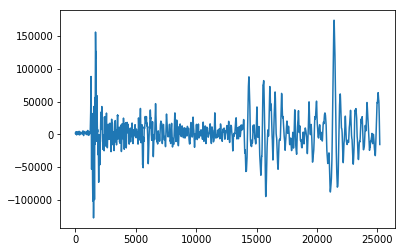Here, plt.plot( ) plots the array EQ against the index number for the elements in the array because we didn't pass a second argument.

We can decorate this plot in many ways. For example, we can add axis labels and truncate the data, or change the color of the line to name a few:

In :
plt.plot(EQ,'r-') # plots as a red line
plt.xlabel('Arbitrary Time') # puts a label on the X axis
plt.ylabel('Velocity'); # puts a label on the Y axis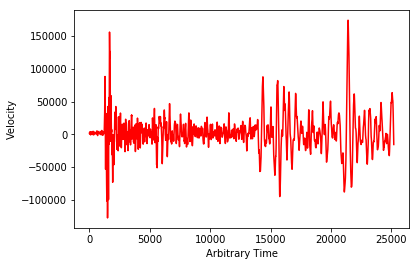### Assignment #2¶

• Make a notebook and change the name of the notebook to: YourLastNameInitial_HW_02 (for example, CychB_HW_02)
• In a markdown cell, write a description of what the notebook does
• Create a list of numbers from 0 to 100
• Create another list that is empty
• Write a for loop that takes the square root of all the values in your list of numbers (using np.sqrt) and appends them to the empty list.
• Print out all the numbers that are divisible by 4 (using the modulo operator).
• Plot the square roots against the original list.
• Create a dictionary with at least 4 key:value pairs

• Write your own module that contains at least four functions and uses a dictionary and a list. Include a doc string in your module and a comment before each function that briefly describes the function. Save it with the magic command %%writefile YOURMODULENAME.py

• Import the module into your notebook and call all of the functions.

Your code must be fully commented.